# 1 Right-angled triangles

An unbroken piece of a circumference of a circle is called an arc. The angle at the centre is said to be subtended by the arc.

One radian is the angle subtended at the centre of a circle by an arc that has the same length as the radius.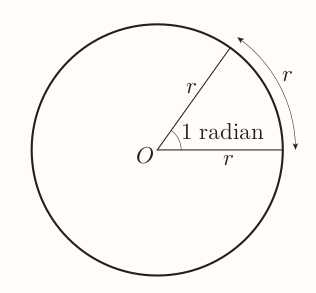Given the circumference of a circle is $2\pi r$ and each arch of length $r$ subtends 1 radian then the number of radians in full circle is: $$\frac{2\pi r}{r} = 2\pi = 360^{\circ}$$ This gives: $$1~\text{radian} = \frac{360^{\circ}}{2\pi} = \frac{180^{\circ}}{\pi} = 57.295... \approx 57^\circ$$

The number of radians in a fraction of full turn can be expressed in terms of $\pi$, e.g. $120^{\circ}= 2\pi/3$, $30^{\circ}= 2\pi/12 = \pi/6$, $90^\circ = \pi/2$...

#### Converting between degrees and radians

• number of radians $= \frac{\pi}{180} \times$ number of degrees
• number of degrees $= \frac{180}{\pi} \times$ number of radians

#### Length of an arc of a circle

$$\text{arc length} = r\theta$$ where $r$ is the radius of the circle and $\theta$ is the angle subtended by the arc, measured in radians.

#### Area of a sector of a circle

$$\text{area of sector} = \frac{1}{2}r^2\theta$$

## 1.2 Sine, cosine and tangent

\begin{align} \sin\theta = \frac{\text{opp}}{\text{hyp}} \\[1em] \cos\theta = \frac{\text{adj}}{\text{hyp}} \\[1em] \tan\theta = \frac{\text{opp}}{\text{adj}} \\[1em] \end{align}

## 1.5 Trigonometric rations of special angles

Following special angles can be deduced without calculator:

• $\pi / 6 ~~ (30^{\circ})$
• $\pi / 4 ~~ (45^{\circ})$
• $\pi / 3 ~~ (60^{\circ})$

$\pi / 3$ is one of the angles of an equilateral triangle like: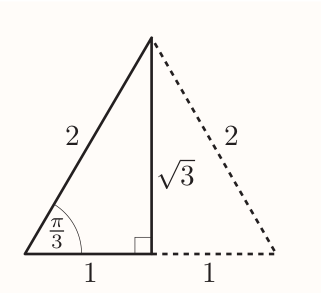The lengths of the vertical line is: $$\sqrt{2^2 - 1^2} = \sqrt{3}$$ Giving: $$\sin\frac{\pi}{3} = \frac{\sqrt{3}}{2} ~~~ \cos\frac{\pi}{3} = \frac{1}{2} ~~~ \tan\frac{\pi}{3} = \frac{\sqrt{3}}{1} = \sqrt{3}$$

The ratio of $\pi/6$ can be found by using same diagram: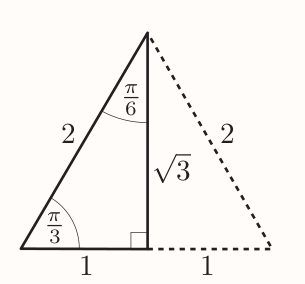Giving: $$\sin\frac{\pi}{6} = \frac{1}{2} ~~~ \cos\frac{\pi}{6} = \frac{\sqrt{3}}{2} ~~~ \tan\frac{\pi}{6} = \frac{1}{\sqrt{3}}$$

To find the ratios of $\pi/4$, following diagram can be used: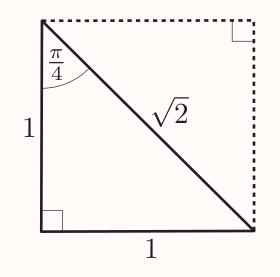The length of the diagonal is: $$\sqrt{1^2 + 1^2} = \sqrt{2}$$

Giving: $$\sin\frac{\pi}{4} = \frac{1}{\sqrt{2}} ~~~ \cos\frac{\pi}{4} = \frac{1}{\sqrt{2}} ~~~ \tan\frac{\pi}{4} = \frac{1}{1} = 1$$

#### Special angles table

$\theta$ in radians$\theta$ in degrees$\sin\theta$$\cos\theta$$\tan\theta$
$\frac{\pi}{6}$$30^{\circ}$$\frac{1}{2}$$\frac{\sqrt{3}}{2}$$\frac{1}{\sqrt{3}}$
$\frac{\pi}{4}$$45^{\circ}$$\frac{1}{\sqrt{2}}$$\frac{1}{\sqrt{2}}$$1$
$\frac{\pi}{3}$$60^{\circ}$$\frac{\sqrt{3}}{2}$$\frac{1}{2}$$\sqrt{3}$

It follows that e.g. $$\cos^{-1}(\frac{\sqrt{3}}{2}) = \frac{\pi}{6}$$

It also follows that for any acute angle $\theta$, the value of $\sin\theta$ and $\cos\theta$ is less than 1.

## 1.6 Trigonometric identities

To find first two identities consider an acute angle $\theta$ in right-angled triangle such that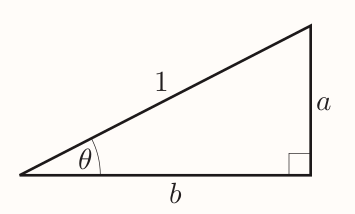For which $$\sin\theta = \frac{a}{1} = a , \cos\theta = \frac{b}{1} = b , \tan\theta = \frac{a}{b}$$

It follows that $$\frac{\sin\theta}{\cos\theta} = \frac{a}{b} = \tan\theta$$ Giving the first identity.

Second identity can be found by applying the Pythagora's theorem: $$a^2 + b^2 = 1^2 = 1$$ Hence it follows that $$(\sin\theta)^2 + (\cos\theta)^2 = 1$$ Giving the second identity.

For next two identities consider: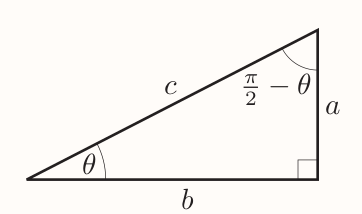Where: $$\sin\theta=\frac{a}{c} ~~ \cos\theta=\frac{b}{c}$$ Leading to next two identities: $$\sin(\frac{\pi}{2} - \theta) = \frac{b}{c} = \cos\theta ~~ \cos(\frac{\pi}{2} - \theta) = \frac{a}{c} = \sin\theta$$

#### List of trigonometric identities

\begin{align} \frac{\sin\theta}{\cos\theta} &= \tan\theta \\[1em] \sin^2\theta + \cos^2\theta &= 1 \\[1em] \sin(\frac{\pi}{2} - \theta) &= \cos\theta \\[1em] \cos(\frac{\pi}{2} - \theta) &= \sin\theta \\[1em] \end{align}

# 2 Trigonometric functions

## 2.1 Angles of any size

Besides acute and right angles we can have:

• obtuse angles (between $\pi/2$ and $\pi$)
• straight angles ($\pi$)
• reflex angles (between $\pi$ and $2\pi$)

There can also be angles larger than $2\pi$ or negative angles like $-\pi$.

The first step to find trigonometric rations of any angle is to associate a particular point $P$ with angle $\theta$ on the unit circle.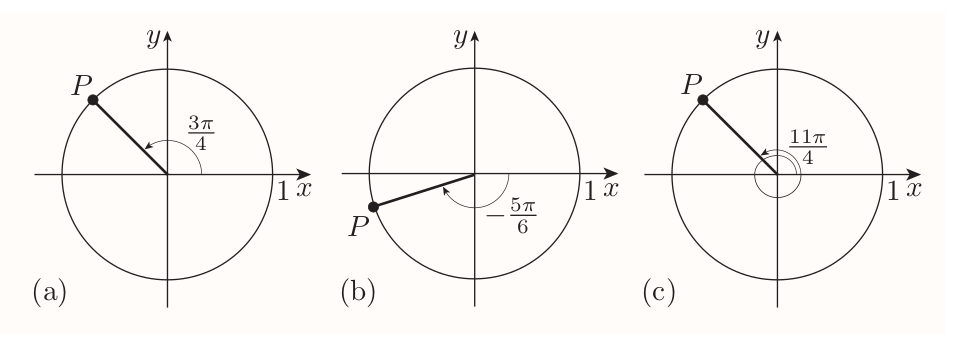## 2.2 Sine, cosine and tangent of any angle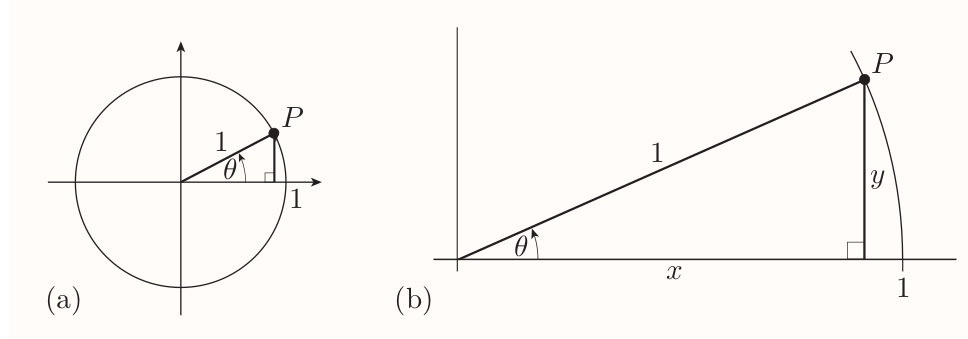#### Sine, cosine and tangent

Suppose that $\theta$ is any angle and $(x, y)$ are the coordinates of its associated point $P$ on the unit circle. Then $$\sin\theta = y~,~~ \cos\theta = x, \\$$ and, provided that $x \ne 0$, $$\tan\theta = \frac{y}{x}$$ (If $x = 0$, then $\tan\theta$ is undefined.)

#### ASTC diagram

Indicates which of the $\sin\theta$, $\cos\theta$ and $\tan\theta$ are positive.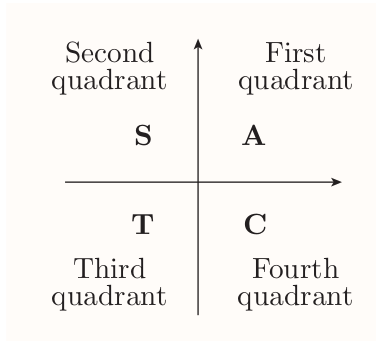Suppose that $\theta$ is an angle whose associated point $P$ does not lie on either the $x$- or $y$-axis, and $\phi$ is the acute angle between $OP$ and the $x$-axis. Then \begin{align} \sin\theta = \pm\sin\phi \\[0.5em] \cos\theta = \pm\cos\phi \\[0.5em] \tan\theta = \pm\tan\phi \\[0.5em] \end{align} The ASTC diagram tells you which sign applies in each case. (The values $\sin\phi$, $\cos\phi$ and $\tan\phi$ are all positive, because $\phi$ is acute.)

## 2.3 Graphs of sine, cosine and tangent

Sine, cosine and tangent are trigonometric functions (rules with inputs and outputs).

### The graph of the sine function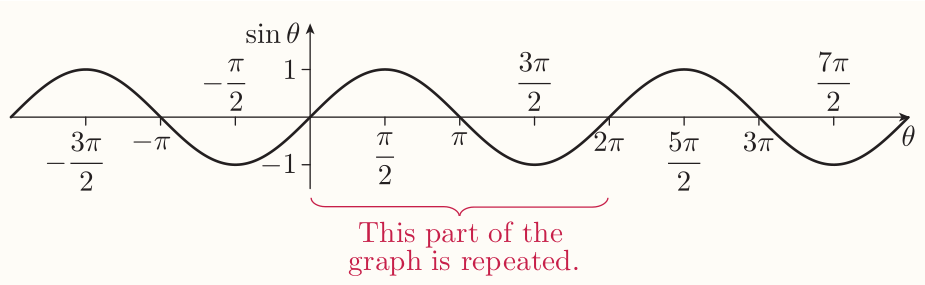$\sin\theta$ is the $y$-coordinate of point $P$ on unit circle. As the angle $\theta$ increases from $0$ to $2\pi$, the point $P$ rotates from positive $x$-axis. Its $y$-coordinate oscillates from 0 to 1 to 0 to -1 and back to 0.

The graph oscillates endlessly since $\sin(\theta + 2\pi) = \sin\theta$ hence it's periodic with period $2\pi$.

### The graph of the cosine function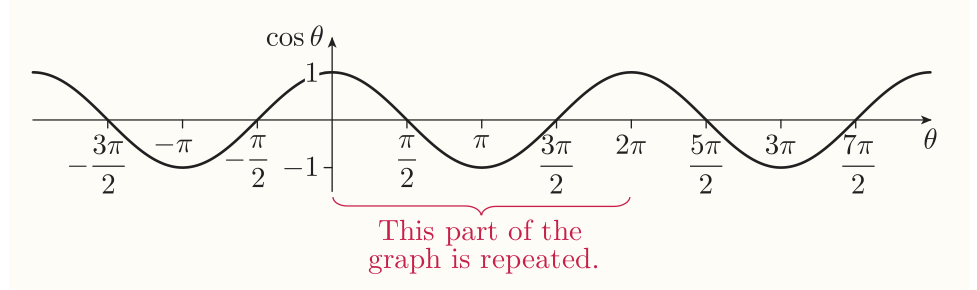$\cos\theta$ is the $x$-coordinate of the point $P$ on the unit circle. It's periodic with period $2\pi$.

### The graph of the tangent function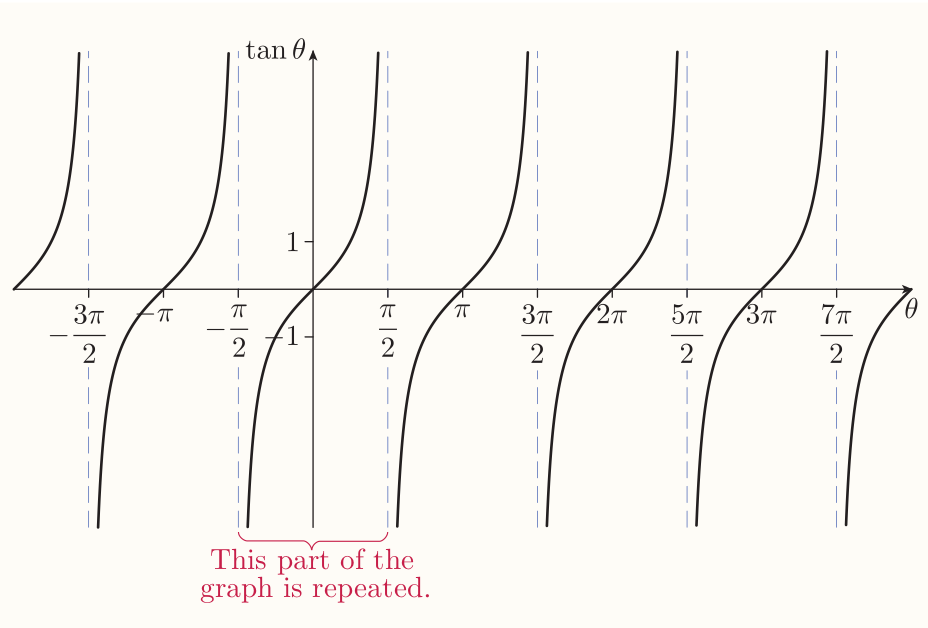The graph is periodic with period of $\pi$. The breaks in graph occur when $\theta = \pi/2 + n\pi$ for some integer $n$. These are the values of $\theta$ for which $\tan\theta$ is undefined because the $x$-coordinate of the point $P$ is zero.

### Using the variables $x$ and $y$ for trigonometric functions

Sometimes it's convenient to use the letter $x$ to denote the input variable and the letter $y$ to denote the output variable:

$$y = \sin x ~~ y = \cos x ~~ y = \tan x$$

## 2.4 Inverse trigonometric functions

In general if a function $f$ is one-to-one, then it has an inverse function $f^{-1}$, whose rule is give by $$f^{-1}(y) = x ~~~\text{where}~~~ f(x) = y$$ The sine, cosine and tangent aren't one-to-one, e.g.: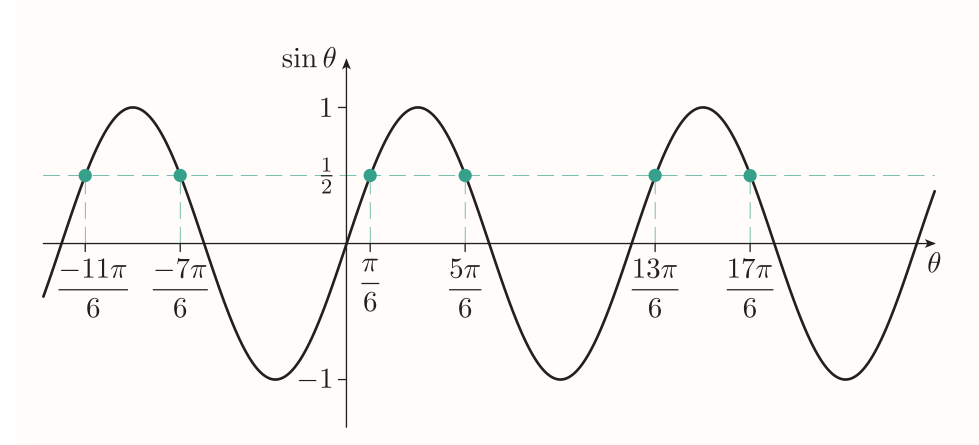To obtain 'sort of inverse function' we need to specify new function with same rule but limited domain - a restriction: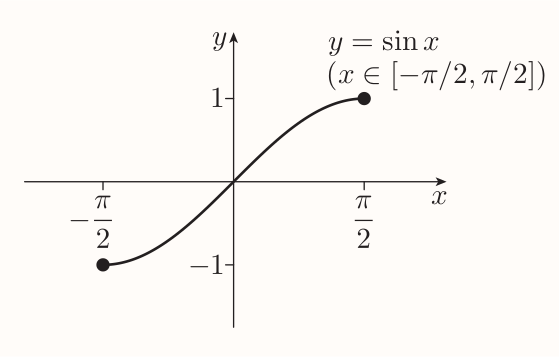It has same image set as the sine function $[-1, 1]$ and it's increasing on the whole domain, so it's one-to-one. Hence it has an inverse function called the inverse sine function denoted by $\sin^{-1}$.

The graph of $y = \sin^{-1}(x)$ is reflection of $y = \sin(x)$ in the line $y = x$ (as with any other inverse function).

### Inverse sine

The inverse sine function $\sin^{-1}$ is the function with domain $[-1, 1]$ and rule $$\sin^{-1}x = y$$ where $y$ is the number in the interval $[-\pi/2, \pi/2]$ such that $\sin y=x$.

### Inverse cosine

The inverse cosine function $\cos^{-1}$ is the function with domain $[-1, 1]$ and rule $$\cos^{-1} x = y$$ where $y$ is the number in the interval $[0, \pi]$ such that $\cos y = x$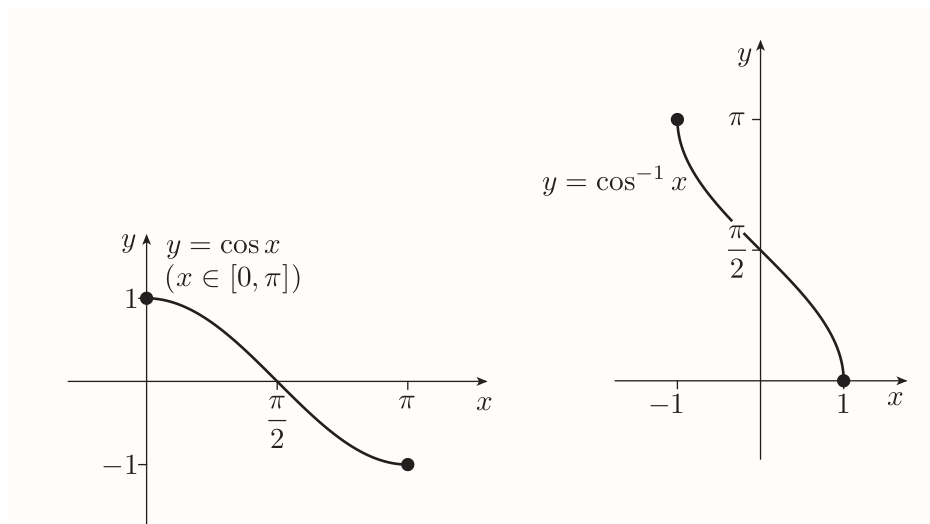### Inverse tangent

The inverse tangent function $\tan^{-1}$ is the function with domain $\mathbb{R}$ and rule $$\tan^{-1} x = y$$ where $y$ is the number in the interval $(-\pi/2, \pi/2)$ such that $\tan y = x$.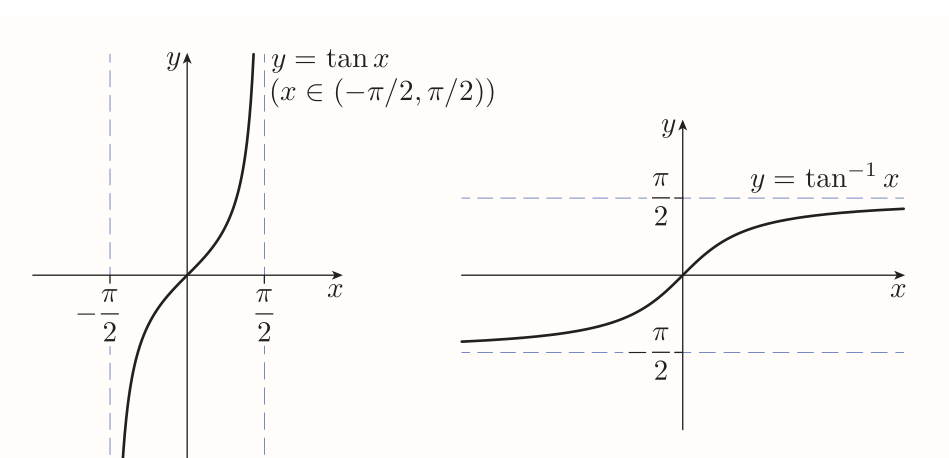#### Identities with negative inputs

\begin{align} \sin^{-1}(-x) &= -\sin^{-1}x \\[0.4em] \cos^{-1}(-x) &= \pi -\cos^{-1}x \\[0.4em] \tan^{-1}(-x) &= -\tan^{-1}x \\[0.4em] \end{align}

## 2.5 Solving simple trigonometric equations

Like: $$\sin\theta=\frac{1}{2},~~~\cos\theta=0.3,~~~\tan\theta=-1$$ One solution can be found by applying an inverse trigonometric function: $$\theta = \sin^{-1}\frac{1}{2} = \frac{\pi}{6}$$ However there are infinitely many other solutions since the sine is periodic. Hence it's the best to start by finding all the solutions in the interval of length $2\pi$, such as $[0, 2\pi]$ or $[-\pi, \pi]$ (there are usually two). Additional solutions ca be found by adding integer multiples of $2\pi$.

# 3 Sine and cosine rules

## 3.1 The sine rule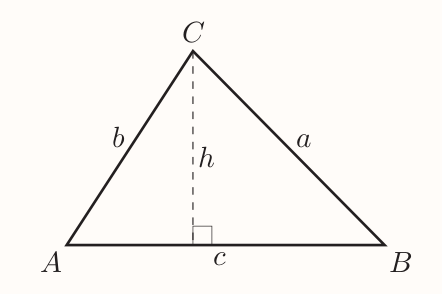\begin{align} \sin A &= \frac{h}{b}, \text{so} h = b\sin A \\[0.4em] \sin B &= \frac{h}{a}, \text{so} h = a\sin B \\[0.4em] \text{hence} \\[0.4em] b\sin A &= h = a \sin B \\[0.4em] \frac{a}{\sin A} &= \frac{b}{\sin B} \end{align}

In same way, the line $h$ can be raised from other vertices and similar equation deduced.

### Sine rule

$$\frac{a}{\sin A} = \frac{b}{\sin B} = \frac{c}{\sin C}$$

### Using the sine rule to find a side length

You can use the sine rule to find an unknown side length of a triangle if you know

• the opposite angle
• another side length and its opposite angle.

### Using the sine rule to find an angle

You can use the sine rule to find an unknown angle in a triangle if you know:

• the opposite side length
• another side length and its opposite angle.

Sometimes you also need to know whether the unknown angle is acute or obtuse.

## 3.2 The cosine rule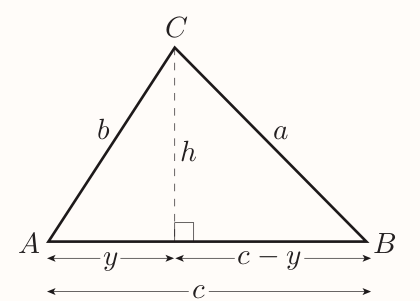\begin{align} b^2 &= y^2 + h^2 \\ a^2 &= (c - y)^2 + h^2 \\ \end{align} Expanding the brackets in second term gives $$a^2 = c^2 - 2cy + y^2 + h^2$$ Since $y^2 + h^2 = b^2$ it follows that $$a^2 = c^2 - 2cy + b^2 = b^2 + c^2 - 2cy$$ Next $$\cos A = \frac{y}{b}, ~~\text{so} ~~ y = b\cos A$$ Substituting gives $$a^2 = b^2 + c^2 - 2bc\cos A$$

#### Cosine rule

\begin{align} a^2 = b^2 + c^2 - 2bc\cos A \\ b^2 = c^2 + a^2 - 2ca\cos B \\ c^2 = a^2 + b^2 - 2ab\cos C \\ \end{align}

### Using the cosine rule to find a side length

You can use the cosine rule to find an unknown side length of a triangle if you know the other two side lengths and the angle between them.

### Using the cosine rule to find an angle

You can use the cosine rule to find an unknown angle if you know all three side lengths.

### Triangle solving tree

graph TD
l1(Does the triangle contain a right angle?)
l2a(Does the problem only involve lengths?)
l2b(Do you know a length and the angle opposite?)
l3a(Pythagoras' theorem)
l3b(Trigonometric ratios)
l3c(Sine rule)
l3d(Cosine rule)

l1 -- Y --> l2a
l1 -- N --> l2b

l2a -- Y --> l3a
l2a -- N --> l3b

l2b -- Y --> l3c
l2b -- N --> l3d


## 3.3 The area of triangle

For a triangle, $$\text{area} = \frac{1}{2} \times \text{base} \times \text{height}$$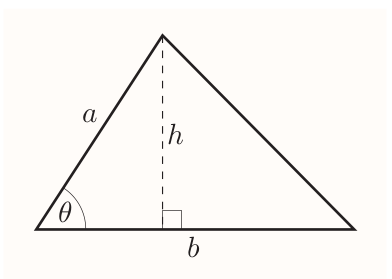$$\sin\theta = \frac{h}{a},~~~\text{so}~~ h = a\sin\theta$$ Hence

### Area of triangle

For a triangle with an angle $\theta$ between two sides of lengths $a$ and $b$, $$\text{area} = \frac{1}{2}ab\sin\theta$$

## 3.4 The angle of inclination of a line

is the angle that it makes with the $x$-axis, measured anticlockwise from the positive direction of the $x$-axis, when the line is drawn on axes with equal scales.

• a line with positive gradient has an angle of inclination between 0 and $\pi/2$
• a line with negative gradient has an angle of inclination between $\pi/2$ and $\pi$.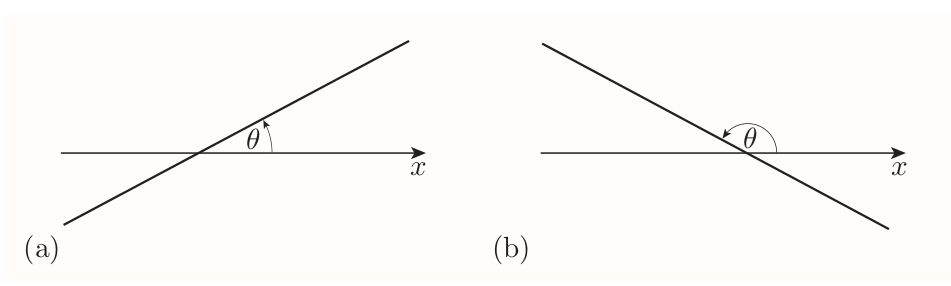When a unit circle is considered: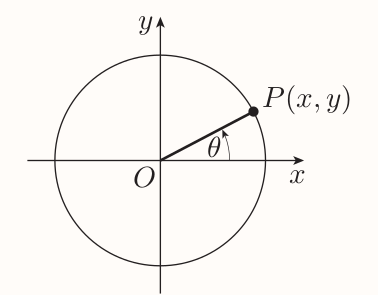It's known that $$\tan\theta = \frac{y}{x}$$ And by the formula for gradient $$\text{gradient of OP} = \frac{y - 0}{x - 0} = \frac{y}{x}$$

hence

### Gradient and angle of inclination of a straight line

For any non-vertical straight line with angle of inclination $\theta$, $$\text{gradient} = \tan\theta$$ Remember that the angle of inclination is measured when the line is drawn on axes with equal scales.

# 4 Further trigonometric identities

## 4.1 Two simple trigonometric identities

First one is already known: $$\tan\theta=\frac{\sin\theta}{\cos\theta}$$

Second can be deduced as follows: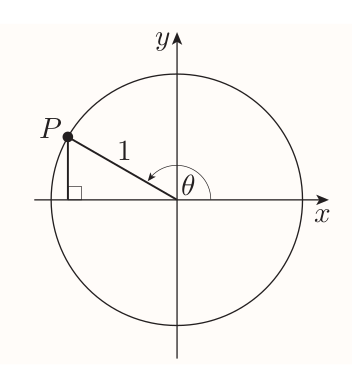The length of the

• vertical side of triangle is $\sin\theta$.
• horizontal side of triangle is $-\cos\theta$

Hence by Pythagoras' theorem \begin{align} (\sin\theta)^2 + (-\cos\theta)^2 = 1^2 \\ (\sin\theta)^2 + (\cos\theta)^2 = 1 \end{align}

Giving the second identity: $$\sin^2\theta + \cos^2\theta = 1$$

## 4.2 Trigonometric identities from the graphs of sine, cosine and tangent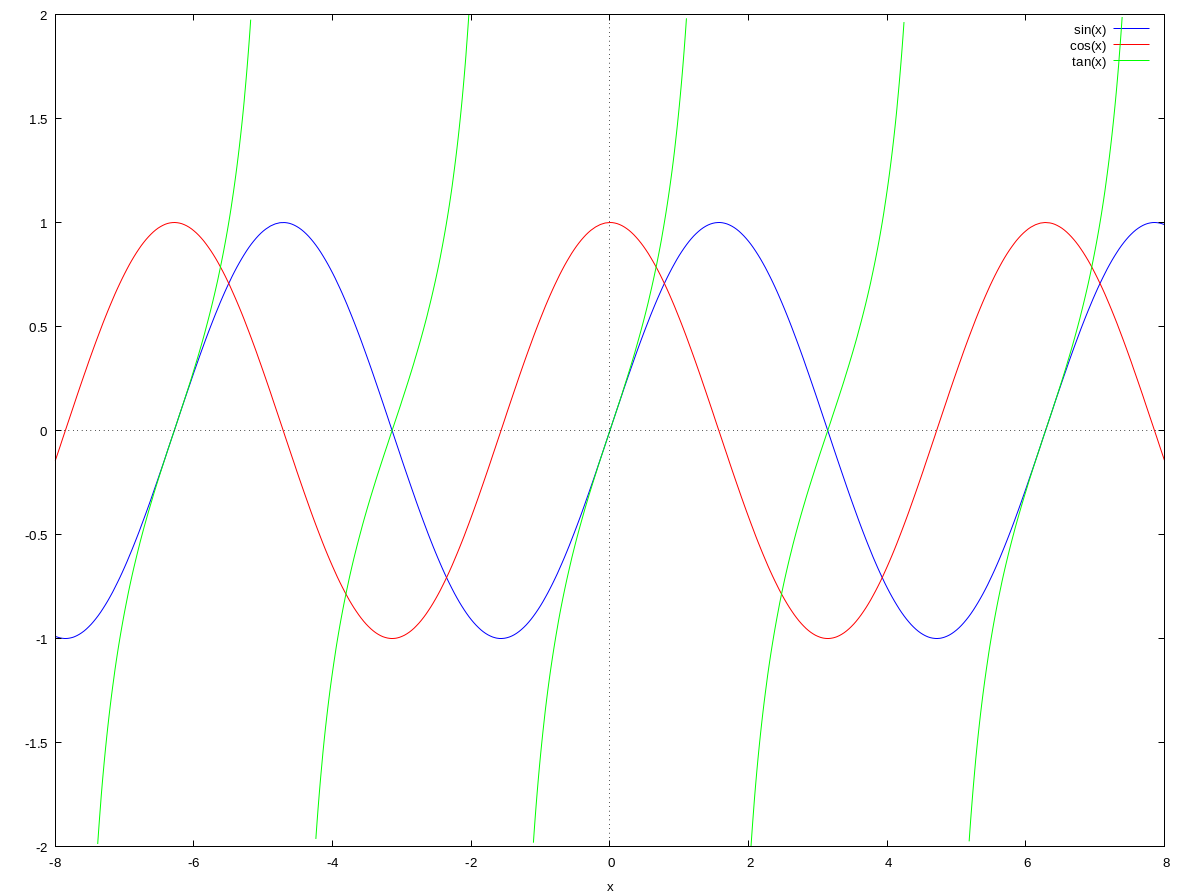Given the trigonometric functions are periodic it follow that

\begin{align} \sin(\theta + 2\pi) &= \sin\theta \\ \cos(\theta + 2\pi) &= \cos\theta \\ \tan(\theta + \pi) &= \tan\theta \\ \end{align}

From the symmetry of the respective graphs it also follows that \begin{align} \sin(-\theta) &= -\sin\theta \\ \cos(-\theta) &= \cos\theta \\ \tan(-\theta) &= -\tan\theta \end{align}

Also, if the graph of cosine is translated to the left by $\pi/2$ and reflected in the vertical axis the graph of sine is obtained. Hence the equation $$\cos(-\theta + \frac{\pi}{2}) = \sin\theta$$ is an identity. Similar identity can be found for since.

Hence following identities \begin{align} \sin(\frac{\pi}{2} - \theta) = \cos\theta \\ \cos(-\theta + \frac{\pi}{2}) = \sin\theta \end{align}

## 4.3 Cosecant, secant and cotangent

In right angled triangle, three known trigonometric functions are defined as $$\sin\theta = \frac{\text{opp}}{\text{hyp}} ~~~ \cos\theta = \frac{\text{adj}}{\text{hyp}} ~~~ \tan\theta = \frac{\text{opp}}{\text{adj}} ~~~$$

There are three further ratios cosecant, secant, cotangent of $\theta$: $$\text{cosec}\theta = \frac{\text{hyp}}{\text{opp}} ~~~ \text{sec}\theta = \frac{\text{hyp}}{\text{adj}} ~~~ \text{cot}\theta = \frac{\text{adj}}{\text{opp}} ~~~$$

Generalized as

### Cosecant, secant and cotangent

\begin{align} \text{cosec}\theta &= \frac{1}{\sin\theta} ~~~(\text{provided} \sin\theta \ne 0) \\ \text{sec}\theta &= \frac{1}{\cos\theta} ~~~(\text{provided} \cos\theta \ne 0) \\ \text{cot}\theta &= \frac{\cos\theta}{\sin\theta} ~~~(\text{provided} \sin\theta \ne 0) \\ \end{align}

Given $$\tan\theta = \frac{\sin\theta}{\cos\theta}$$

it follows that $$\cot\theta = \frac{1}{\tan\theta} ~~~ (\text{provided} \sin\theta\ne0 ~\text{and} \cos\theta\ne0)$$

Essentially cosecant, secant and cotangent are the reciprocals of the sine, cosine and tangent functions (with same periods).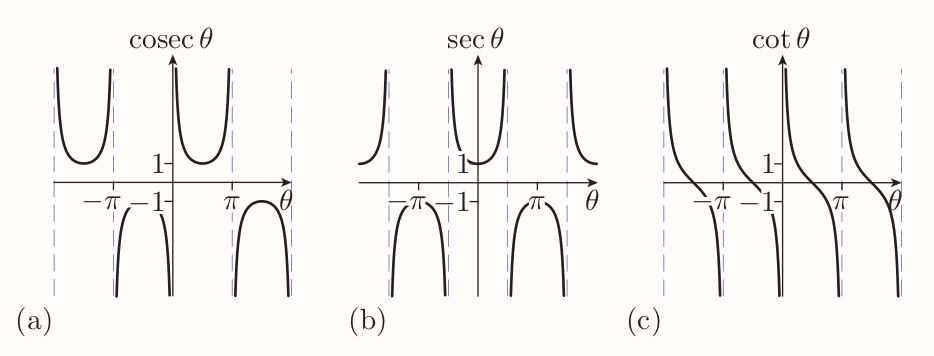Two new identities can be obtained by taking previous identity $$\sin^2\theta + \cos^2\theta = 1$$ dividing both sides by $\cos^2\theta$ $$\frac{\sin^2\theta}{\cos^2\theta} + \frac{\cos^2\theta}{\cos^2\theta} = \frac{1}{\cos^2\theta}$$ that is $$\tan^2\theta + 1 = \sec^2\theta$$

Hence following identities \begin{align} \tan^2\theta + 1 &= \sec^2\theta \\ 1 + \cot^2\theta &= \text{cosec}^2\theta \end{align}

## 4.4 Angle sum and angle difference identities

### Angle sum identities for sine and cosine

\begin{align} \sin(A + B) = \sin A \cos B + \cos A \sin B \\ \cos(A + B) = \cos A \cos B - \sin A \sin B \end{align}

### Angle sum identity for tangent

$$\tan(A + B) = \frac{\tan A + \tan B}{1 - \tan A \tan B}$$

### Angle difference identities

\begin{align} \sin(A + B) = \sin A \cos B - \cos A \sin B \\ \cos(A - B) = \cos A \cos B + \sin A \sin B \\ \tan(A - B) = \frac{\tan A - \tan B}{1 + \tan A \tan B} \end{align}

## 4.5 Double-angle and half-angle identities

### Double-angle identities

\begin{align} \sin(2\theta) &= 2\sin\theta\cos\theta \\ \cos(2\theta) &= \cos^2\theta - sin^2\theta \\ \tan(2\theta) &= \frac{2\tan\theta}{1 - \tan^2\theta} \end{align}

### Alternative double-angle identities for cosine

\begin{align} \cos(2\theta) = 1 - 2\sin^2\theta \\ \cos(2\theta) = 2\cos^2\theta - 1 \end{align}

### Half-angle identities

\begin{align} \sin^2\theta = \frac{1}{2}(1 - \cos(2\theta)) \\ \cos^2\theta = \frac{1}{2}(1 + cos(2\theta)) \end{align}